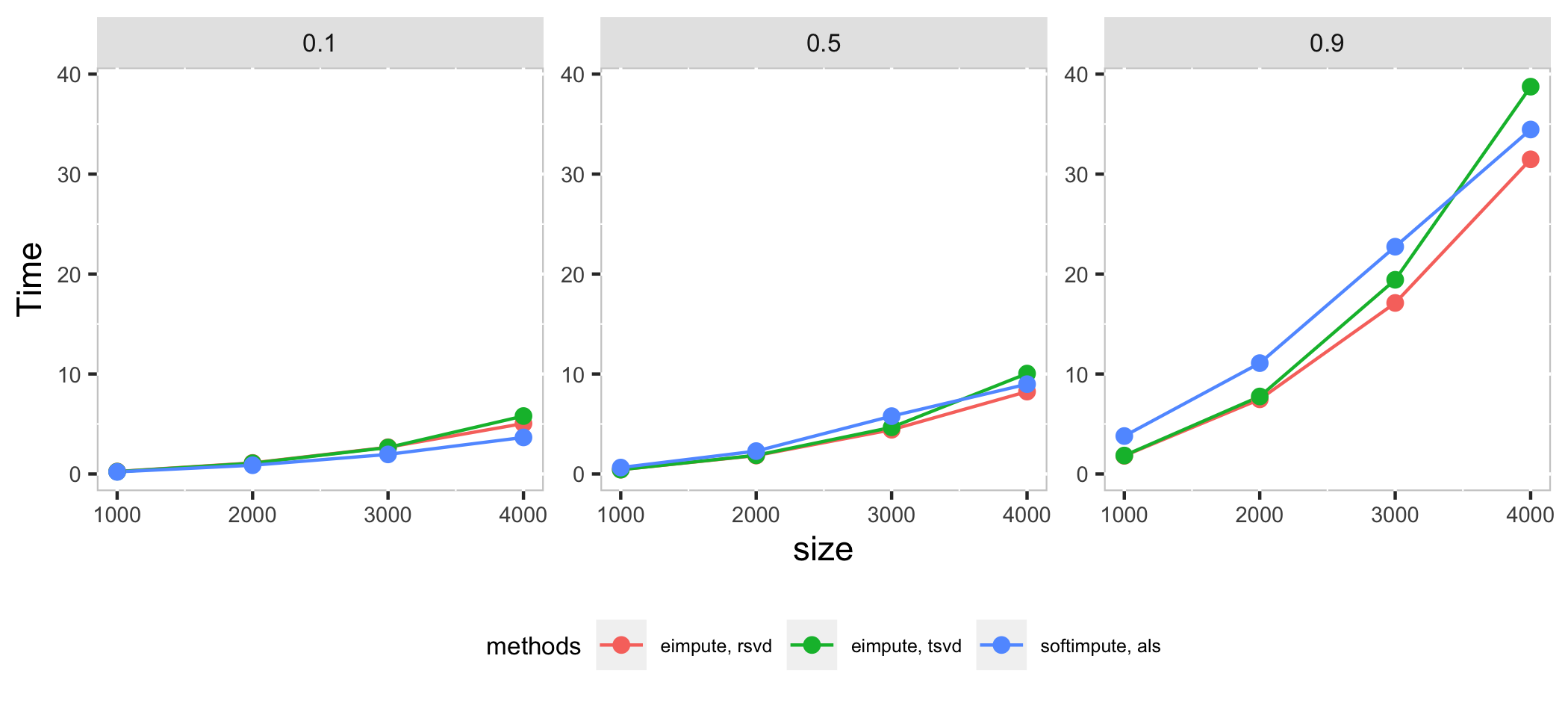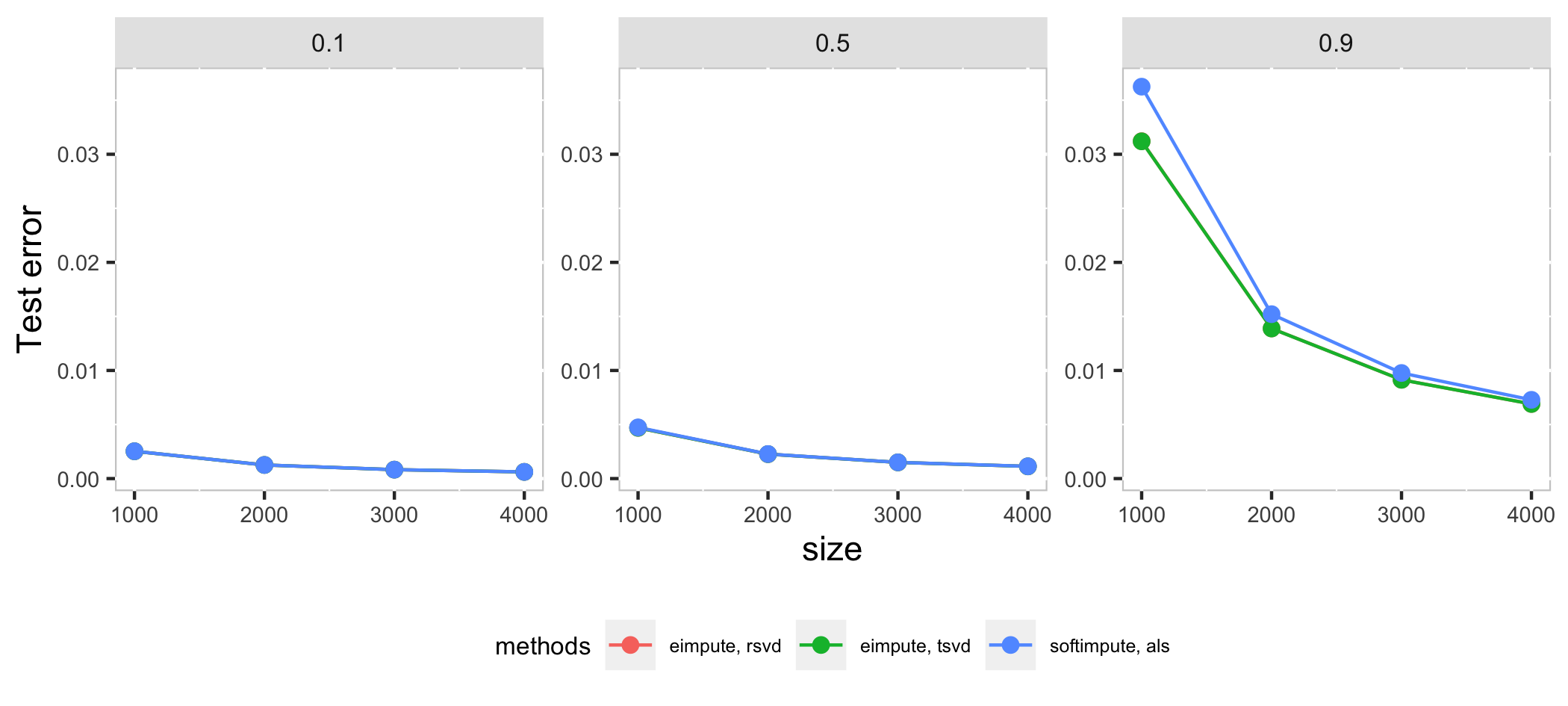# eimpute: Efficiently IMPUTE Large Scale Incomplete Matrix

## Introdution

Matrix completion is a procedure for imputing the missing elements in matrices by using the information of observed elements. This procedure can be visualized as:Matrix completion has attracted a lot of attention, it is widely applied in: - tabular data imputation: recover the missing elements in data table; - recommend system: estimate users’ potantial preference for items pending purchased; - image inpainting: inpaint the missing elements in digit images.

## Software

A computationally efficient R package, eimpute is developed for matrix completion.

### Installation

Install the stable version from CRAN:

install.packages("eimpute")

In eimpute, matrix completion problem is solved by iteratively performing low-rank approximation and data calibration, which enjoy two admirable advantages: - unbiased low-rank approximation for incomplete matrix - less time consumption via truncated SVD Moreover, eimpute also supports flexible data standardization.

Compare eimpute and softimpute in systhesis datasets $$X_{m \times m}$$ with $$p$$ proportion missing observations:

• $$m$$ is chosen as 1000, 2000, 3000, 4000
• $$p$$ is chosen as 0.1, 0.5, 0.9.In high dimension case, als method in softimpute is a little faster than eimpute in low proportion of missing observations, as the proportion of missing observations increase, rsvd method in eimpute have a better performance than softimpute in time cost and test error. Compare with two method in **eimpute*, rsvd method is better than tsvd in time cost.

• Rahul Mazumder, Trevor Hastie and Rob Tibshirani (2010) Spectra Regularization Algorithms for Learning Large Incomplete Matrices, Journal of Machine Learning Research 11 (2010) 2287-2322

• Nathan Halko, Per-Gunnar Martinsson, Joel A. Tropp (2011) Finding Structure with Randomness: Probabilistic Algorithms for Constructing Approximate Matrix Decompositions, SIAM Review Vol. 53, num. 2, pp. 217-288

## Bug report

Send an email to Zhe Gao at gaozh8@mail2.sysu.edu.cn